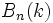# Borel subgroup is abnormal in general linear group

Let$k$ be a field, and$GL_n(k)$ denote the General linear group (?): the group of invertible$n \times n$ matrices over$k$. Let$B_n(k)$ denote the Borel subgroup of$Gl_n(k)$: the subgroup of invertible upper-triangular matrices. Then,$B_n(k)$ is an Abnormal subgroup (?) inside$GL_n(k)$.
Suppose$k$ is a finite field of order$q$, where$q$ is the power of a prime$p$. Then, the Borel subgroup$B_n(k)$ is the normalizer of a$p$-Sylow subgroup of$GL_n(k)$: the subgroup of upper-triangular matrices with$1$s on the diagonal. The abnormality in this case follows from the general fact that the normalizer of a Sylow subgroup is abnormal. Further information: Sylow normalizer implies abnormal